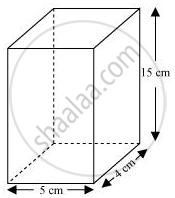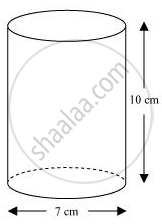# A soft drink is available in two packs − (i) a tin can with a rectangular base of length 5 cm and width 4 cm, having a height of 15 cm and (ii) a plastic cylinder with circular base of diameter 7 cm and height 10 cm. - Mathematics

A soft drink is available in two packs − (i) a tin can with a rectangular base of length 5 cm and width 4 cm, having a height of 15 cm and (ii) a plastic cylinder with circular base of diameter 7 cm and height 10 cm. Which container has greater capacity and by how much?

["Assume "pi=22/7]

#### Solution

The tin can will be cuboidal in shape while the plastic cylinder will be cylindrical in shape.Length (l) of tin can = 5 cm

Breadth (b) of tin can = 4 cm

Height (h) of tin can = 15 cm

Capacity of tin can = l × b × h

= (5 × 4 × 15) cm3

= 300 cm3Radius (r) of circular end of plastic cylinder = (7/2)cm = 3.5 cm

Height (H) of plastic cylinder = 10 cm

Capacity of plastic cylinder = πr2H

=[22/7xx(3.5)^2xx10]cm^3

= (11 x 35) cm3

= 385 cm3

Therefore, plastic cylinder has the greater capacity.

Difference in capacity = (385 − 300) cm= 85 cm3

Concept: Volume of a Cylinder
Is there an error in this question or solution?

#### APPEARS IN

NCERT Class 9 Maths
Chapter 13 Surface Area and Volumes
Exercise 13.6 | Q 3 | Page 230